• Slides: 47포인터(Pointers) v linked representation of ordered lists successive items of a list may be placed anywhere in memory. v associated with each list element is a node which contains both a data component and a pointer, called link, to the next item in the list v ptr 10 node 20 50 Ù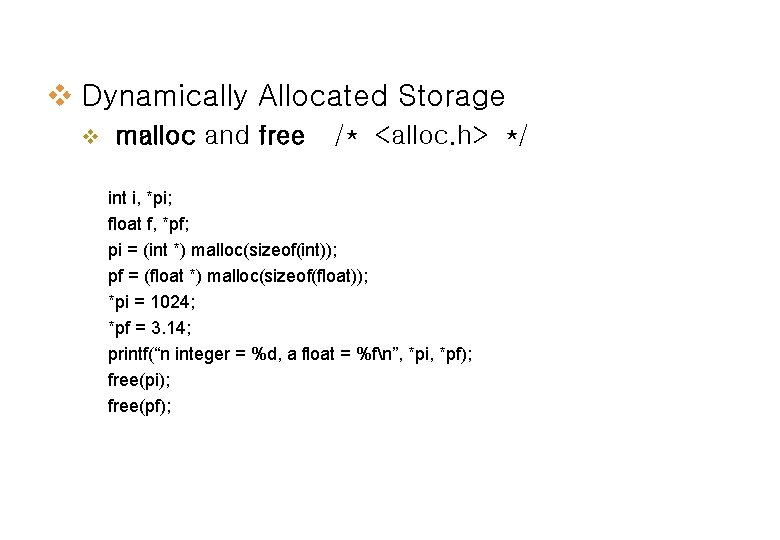v Dynamically Allocated Storage v malloc and free /* <alloc. h> */ int i, *pi; float f, *pf; pi = (int *) malloc(sizeof(int)); pf = (float *) malloc(sizeof(float)); *pi = 1024; *pf = 3. 14; printf(“n integer = %d, a float = %fn”, *pi, *pf); free(pi); free(pf);Singly linked lists v Linked lists representation (1) the nodes do not reside in sequential locations (2) the locations of the nodes may change on different runs ptr bat cat sat vat NULL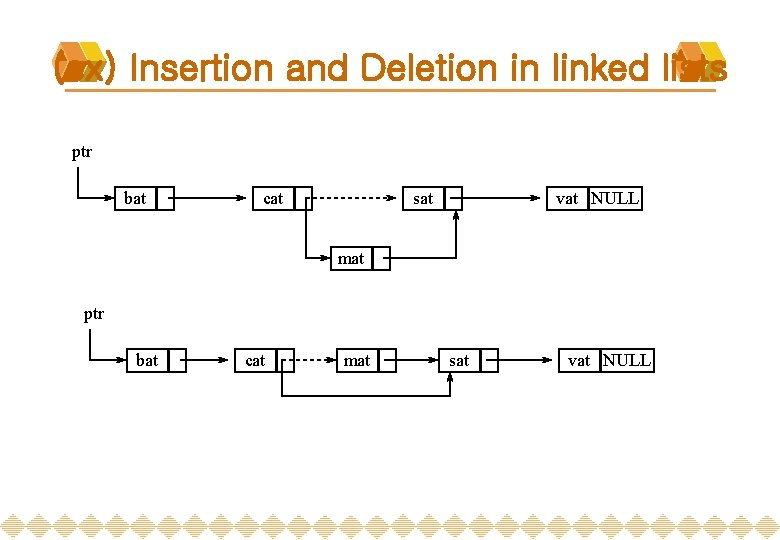(ex) Insertion and Deletion in linked lists ptr bat cat sat vat NULL mat ptr bat cat mat sat vat NULLv Creation of a list Declaration typedef struct list_node *list_pointer; typedef struct list_node { char data ; list_pointer link; struct node{ }; char data; struct node *link; Creation list_pointer ptr =NULL; }; typedef struct node list_node; typedef list_node *list_pointer; Testing #define IS_EMPTY(ptr) (!(ptr)) Allocation ptr=(list_pointer) malloc (sizeof(list_node)); Ÿ Ÿ Ÿ self-referential structure create a pointer to a type that does not get exist NULL: defined in stdio. h(K&RC) or stddef. h(ANSI C)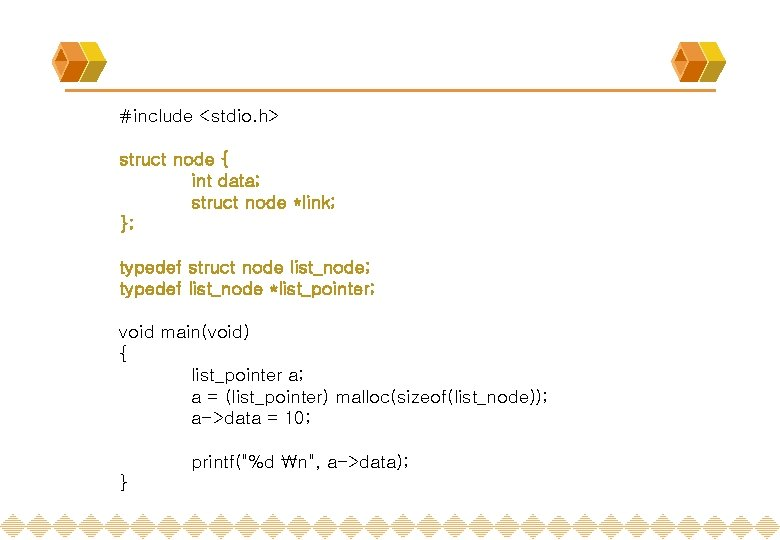#include <stdio. h> struct node { int data; struct node *link; }; typedef struct node list_node; typedef list_node *list_pointer; void main(void) { list_pointer a; a = (list_pointer) malloc(sizeof(list_node)); a->data = 10; printf("%d n", a->data); }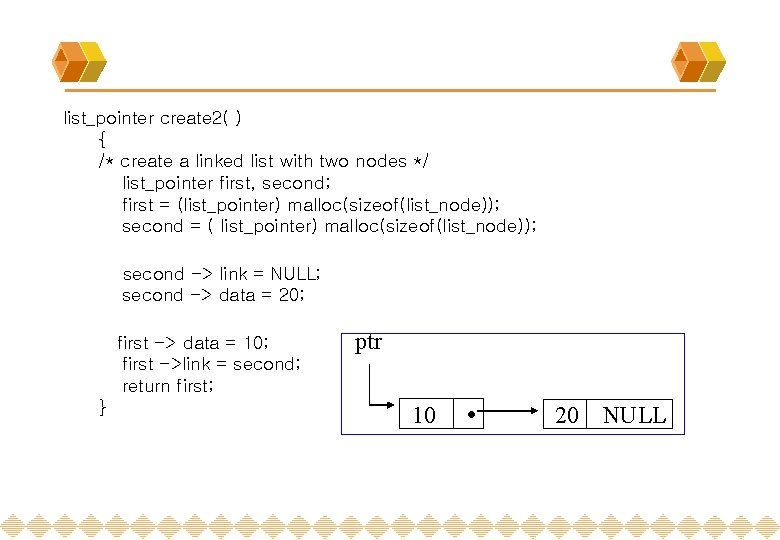list_pointer create 2( ) { /* create a linked list with two nodes */ list_pointer first, second; first = (list_pointer) malloc(sizeof(list_node)); second = ( list_pointer) malloc(sizeof(list_node)); second -> link = NULL; second -> data = 20; first -> data = 10; first ->link = second; return first; } ptr 10 20 NULLint i, *pi; 1000 ? i pi pi = &i; i 1000 ? *pi 2000 pi 1000 i = 10 or *pi = 10 i 1000 10 *pi 2000 pi 1000 2000 ?typedef struct list_node *list_pointer; typedef struct list_node { int data; list_pointer link; }; list_pointer ptr = NULL; ptr 1000 NULL ptr = malloc(sizeof(list_node)); 2000 1000 ptr 2000 data ptr->data� (*ptr). data *ptr link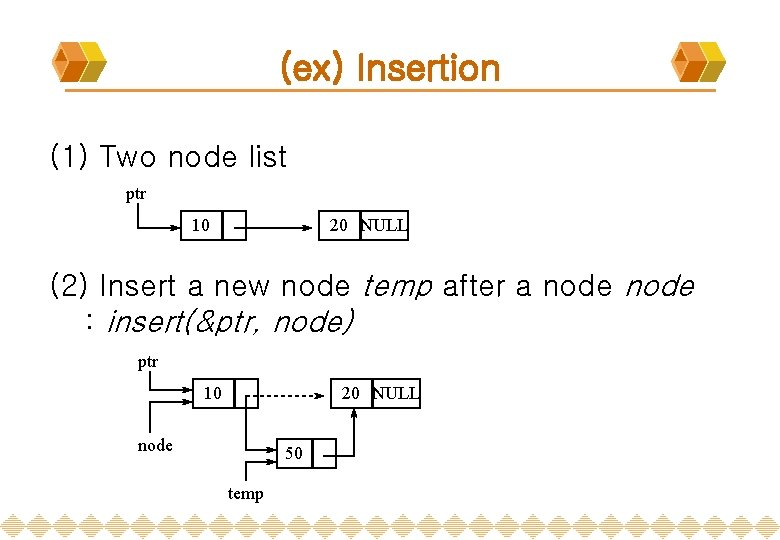(ex) Insertion (1) Two node list ptr 10 20 NULL (2) Insert a new node temp after a node : insert(&ptr, node) ptr 10 20 NULL node 50 temp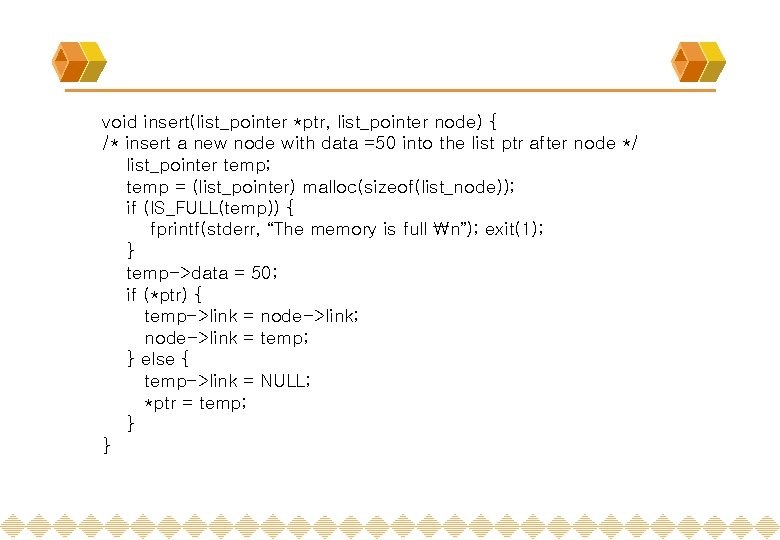void insert(list_pointer *ptr, list_pointer node) { /* insert a new node with data =50 into the list ptr after node */ list_pointer temp; temp = (list_pointer) malloc(sizeof(list_node)); if (IS_FULL(temp)) { fprintf(stderr, “The memory is full n”); exit(1); } temp->data = 50; if (*ptr) { temp->link = node->link; node->link = temp; } else { temp->link = NULL; *ptr = temp; } }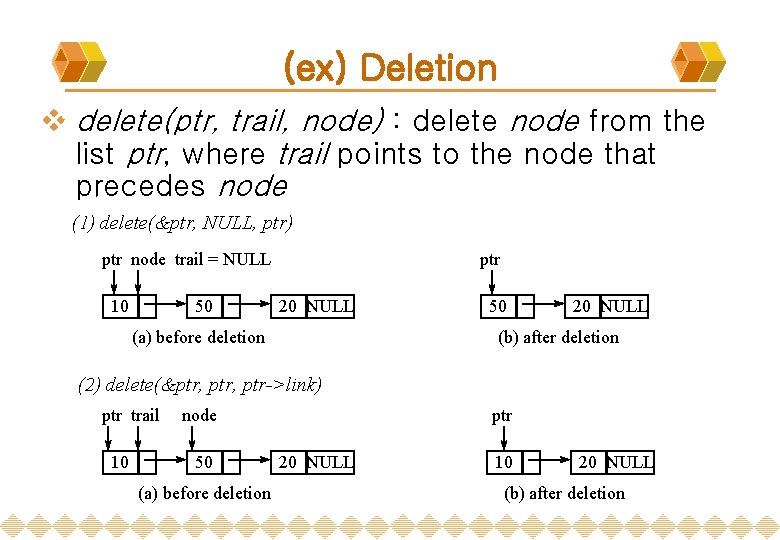(ex) Deletion v delete(ptr, trail, node) : delete node from the list ptr, where trail points to the node that precedes node (1) delete(&ptr, NULL, ptr) ptr node trail = NULL 10 50 ptr 20 NULL (a) before deletion 50 20 NULL (b) after deletion (2) delete(&ptr, ptr->link) ptr trail 10 node 50 (a) before deletion ptr 20 NULL 10 20 NULL (b) after deletionvoid delete (list_pointer *ptr, list_pointer trail, list_pointer node) { /*delete node form the list, trail is the preceding node ptr is the head of the list */ if (trail) trail->link = node->link; else *ptr = (*ptr)->link; free(node); }다항식(Polynomials) v Representing polynomials as linked lists typedef struct poly_node *poly_pointer typedef struct poly_node { int coef; int expon; poly_pointer link; } poly_pointer a, b, d; coef expon linka = 3 x 14 + 2 x 8 + 1 b = 8 x 14 - 3 x 10 + 10 x 6 a 3 14 2 8 1 0 Ù 8 14 -3 10 10 6 Ù bpoly_pointer padd(poly_pointer a, poly_pointer b) { poly_pointer front, rear, temp; int sum; rear =(poly_pointer)malloc(sizeof(poly_node)); if ( IS_FULL(rear) ) { fprintf(stderr, “The memory is fulln”); exit(1); } front = rear; while (a && b) { switch (COMPARE(a->expon, b->expon)) { case -1: /* a->expon < b->expon */ attach(b->coef, b->expon, &rear); b= b->link; break;case 0: /* a->expon == b->expon */ sum = a->coef + b->coef; if (sum) attach(sum, a->expon, &rear); a = a->link; b = b->link; break; case 1: /* a->expon > b->expon */ attach(a->coef, a->expon, &rear); a = a->link; } } for (; a; a = a->link) attach(a->coef, a->expon, &rear); for (; b; b=b->link) attach(b->coef, b->expon, &rear); rear->link = NULL; temp = front; front = front->link; free(temp); return front; }원형리스트(Circular list) v Representing polynomials as circularly linked lists We can free all the nodes more efficiently if we modify our list structure so that the link field of the last node points to the first node in the list, called circular list. v A singly linked list in which the last node has a null link is called a chain. v v Erase a circular list in a fixed amount of time ptr 3 14 2 8 1 0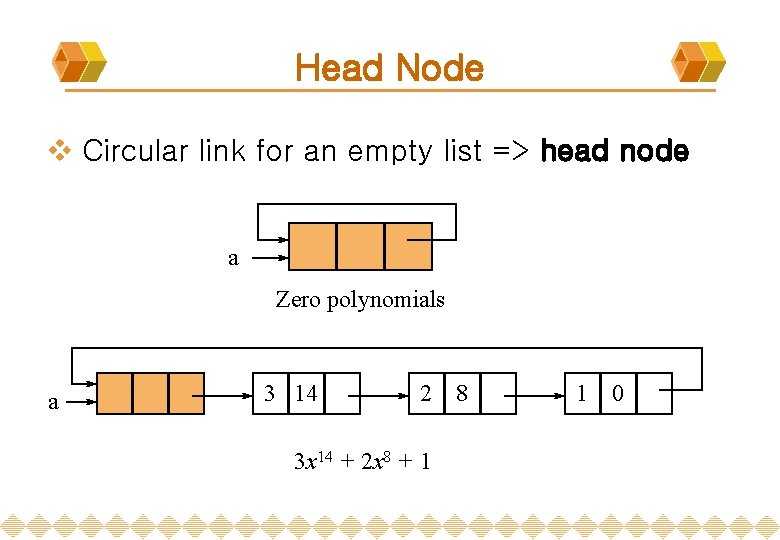Head Node v Circular link for an empty list => head node a Zero polynomials a 3 14 2 3 x 14 + 2 x 8 + 1 8 1 0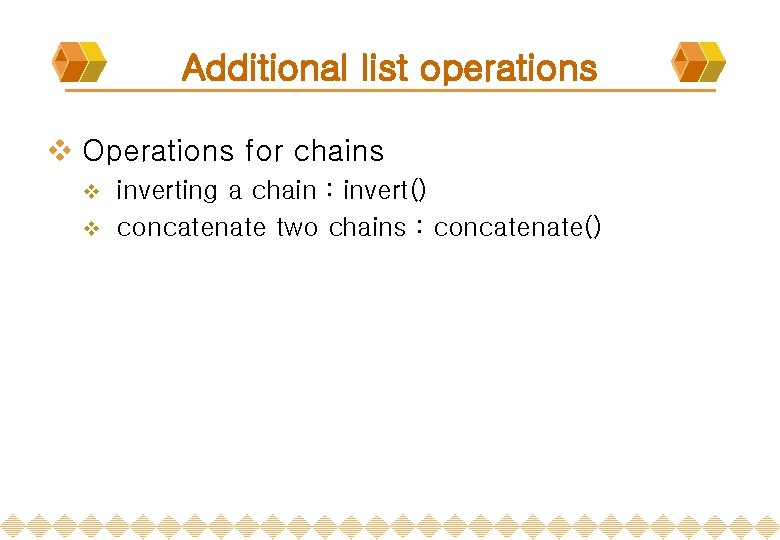Additional list operations v Operations for chains inverting a chain : invert() v concatenate two chains : concatenate() v연결리스트의 역순화 void invert(list_pointer *grocery) { list_pointer *p, *q, *r; p = grocery; q = NULL: while(p != NULL) { r = q; q = p; p = p -> link; q -> link = r; } grocery = q; } struct node { int data; struct node *link; }; typedef struct node list_node; typedef list_node *list_pointer;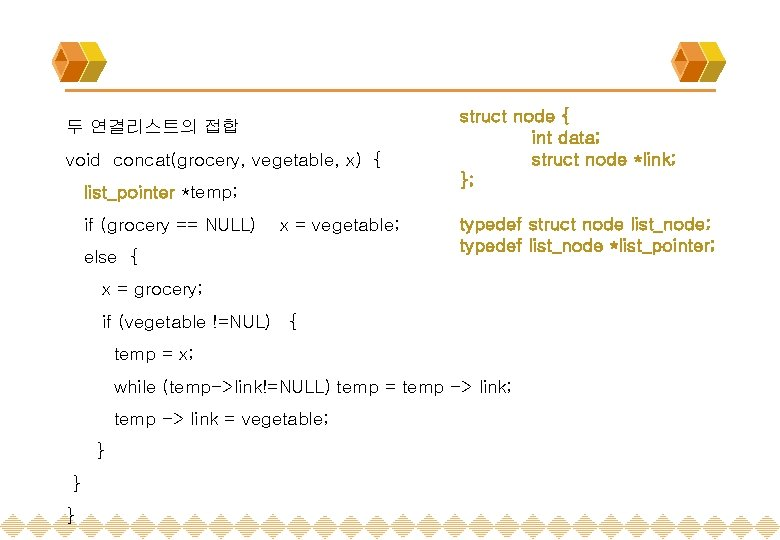두 연결리스트의 접합 void concat(grocery, vegetable, x) { list_pointer *temp; if (grocery == NULL) x = vegetable; else { struct node { int data; struct node *link; }; typedef struct node list_node; typedef list_node *list_pointer; x = grocery; if (vegetable !=NUL) { temp = x; while (temp->link!=NULL) temp = temp -> link; temp -> link = vegetable; } } }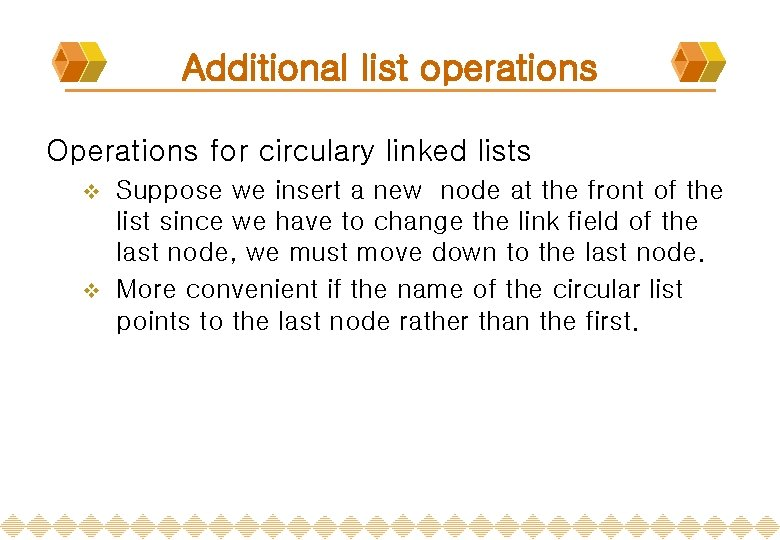Additional list operations Operations for circulary linked lists Suppose we insert a new node at the front of the list since we have to change the link field of the last node, we must move down to the last node. v More convenient if the name of the circular list points to the last node rather than the first. v(ex) Circular list with the name pointing to the last node (a) point to the first node x 1 x 2 xn ptr (b) point to the last node x 1 x 2 xn ptr v Insertion of a node at the front or at the rear of a circular list takes a fixed amount of time.Sparse Matrices inadequacies of sequential schemes (1) # of nonzero terms will vary after some matrix computation (2) matrix just represents intermediate results new scheme Each column (row): a circular linked list with a head nodeRevisit Sparse Matrices # of head nodes = max{# of rows, # of columns} head node entry node down head right next down entry row col value aij entry i aij j right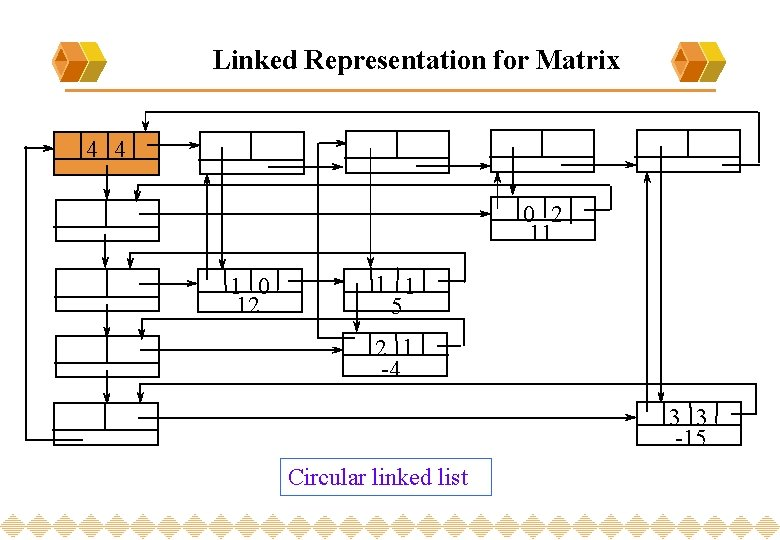Linked Representation for Matrix 4 4 0 2 11 1 0 12 1 1 5 2 1 -4 3 3 -15 Circular linked list#define MAX_SIZE 50 /* size of largest matrix */ typedef enum {head, entry} tagfield; typedef struct matrix_node *matrix_pointer; typedef struct entry_node { int row; int col; int value; }; typedef struct matrix_node { matrix_pointer down; matrix_pointer right; tagfield tag; union { matrix_pointer next; entry_node entry; } u; }; matrix_pointer hdnode[MAX_SIZE];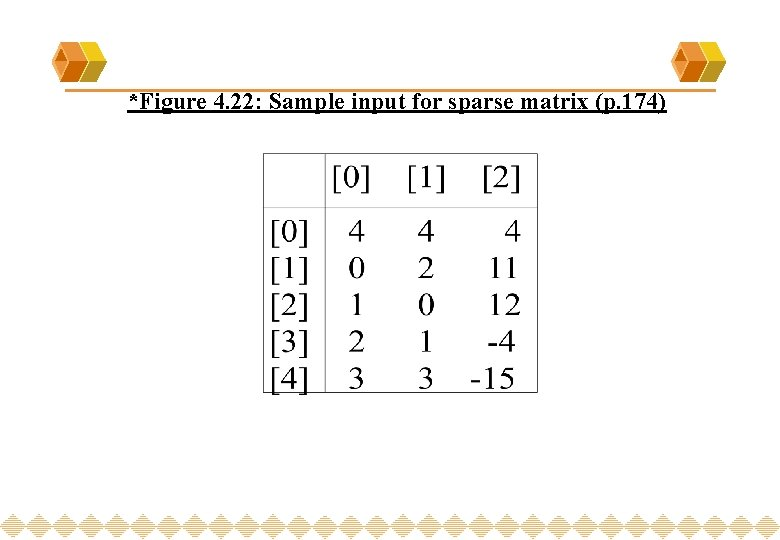*Figure 4. 22: Sample input for sparse matrix (p. 174)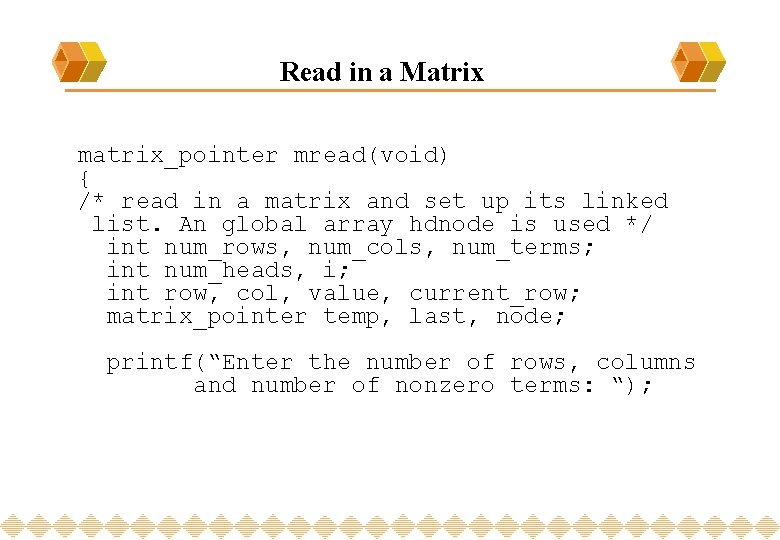Read in a Matrix matrix_pointer mread(void) { /* read in a matrix and set up its linked list. An global array hdnode is used */ int num_rows, num_cols, num_terms; int num_heads, i; int row, col, value, current_row; matrix_pointer temp, last, node; printf(“Enter the number of rows, columns and number of nonzero terms: “);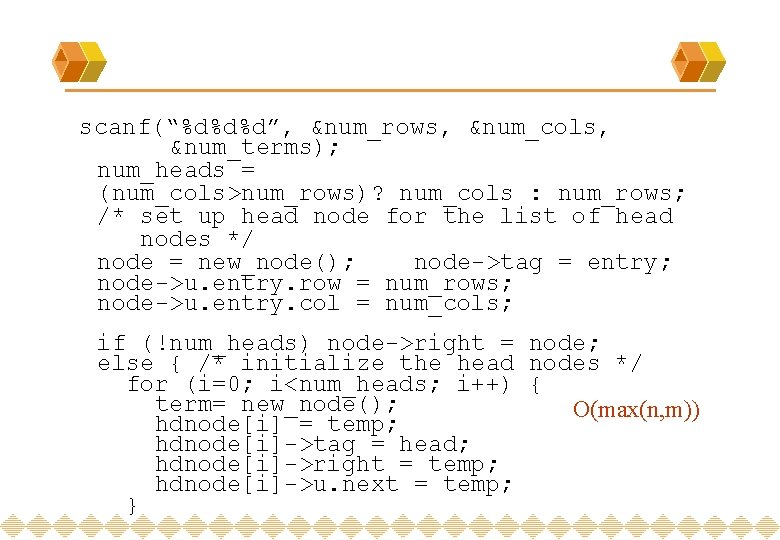scanf(“%d%d%d”, &num_rows, &num_cols, &num_terms); num_heads = (num_cols>num_rows)? num_cols : num_rows; /* set up head node for the list of head nodes */ node = new_node(); node->tag = entry; node->u. entry. row = num_rows; node->u. entry. col = num_cols; if (!num_heads) node->right = node; else { /* initialize the head nodes */ for (i=0; i<num_heads; i++) { term= new_node(); O(max(n, m)) hdnode[i] = temp; hdnode[i]->tag = head; hdnode[i]->right = temp; hdnode[i]->u. next = temp; }current_row= 0; last= hdnode; for (i=0; i<num_terms; i++) { printf(“Enter row, column and value: ”); scanf(“%d%d%d”, &row, &col, &value); if (row>current_row) { last->right= hdnode[current_row]; current_row= row; last=hdnode[row]; } temp = new_node(); temp->tag=entry; temp->u. entry. row=row; temp->u. entry. col = col; temp->u. entry. value = value; last->right = temp; /*link to row list */ last= temp; /* link to column list */ hdnode[col]->u. next->down = temp; hdnode[col]=>u. next = temp; }/*close last row */ last->right = hdnode[current_row]; /* close all column lists */ for (i=0; i<num_cols; i++) hdnode[i]->u. next->down = hdnode[i]; /* link all head nodes together */ for (i=0; i<num_heads-1; i++) hdnode[i]->u. next = hdnode[i+1]; hdnode[num_heads-1]->u. next= node; node->right = hdnode; } } return node; O(max{#_rows, #_cols}+#_terms)Write out a Matrix void mwrite(matrix_pointer node) { /* print out the matrix in row major form */ int i; matrix_pointer temp, head = node->right; printf(“n num_rows = %d, num_cols= %dn”, node->u. entry. row, node->u. entry. col); printf(“The matrix by row, column, and value: nn”); for (i=0; i<node->u. entry. row; i++) { for (temp=head->right; temp!=head; temp=temp->right) printf(“%5 d%5 d%5 dn”, temp->u. entry. row, temp->u. entry. col, temp->u. entry. value); head= head->u. next; /* next row */ } }Erase a Matrix void merase(matrix_pointer *node) { int i, num_heads; matrix_pointer x, y, head = (*node)->right; for(i=0; i<(*node)->u. entry. row; i++) { y=head->right; while (y!=head) { x = y; y = y->right; free(x); } x= head; head= head->u. next; free(x); } y = head; while (y!=*node) { x = y; y = y->u. next; free(x); } free(*node); *node = NULL; } O(#_rows+#_cols+#_terms)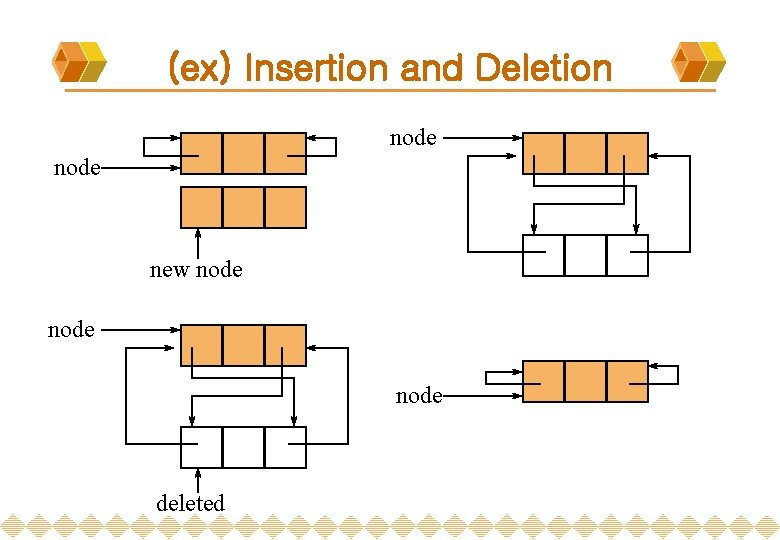(ex) Insertion and Deletion node new node deleted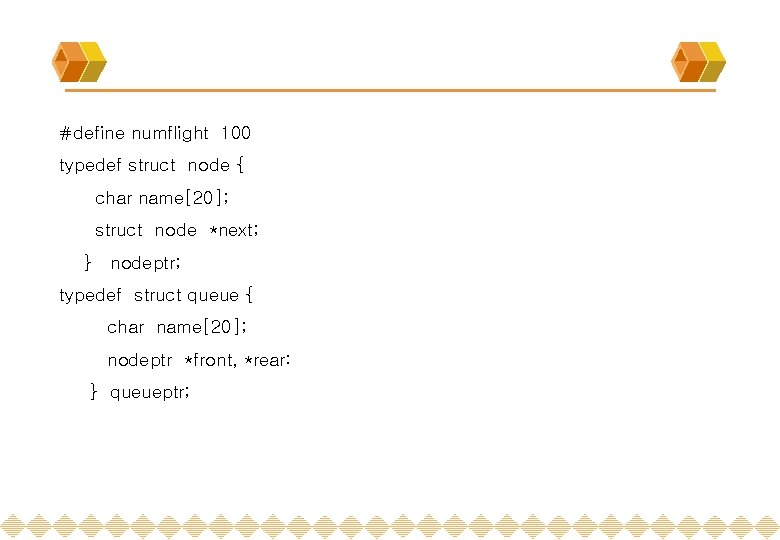#define numflight 100 typedef struct node { char name; struct node *next; } nodeptr; typedef struct queue { char name; nodeptr *front, *rear: } queueptr;** 비행기 예약 구조체 typedef struct flighttype { char fltno; int capacity; int count; nodeptr *flthead; queueptr *waitlist; } flight; Flight flight[numflight];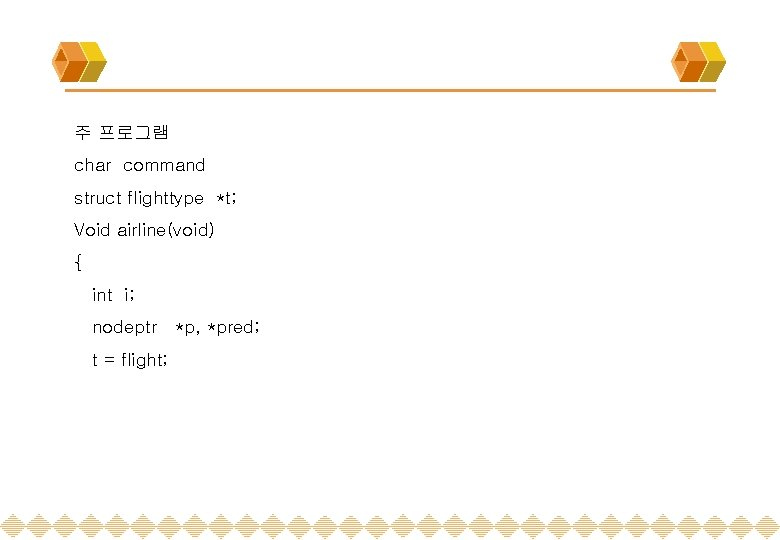주 프로그램 char command struct flighttype *t; Void airline(void) { int i; nodeptr *p, *pred; t = flight;** 초기화 For (i=0; i < numflight; i++) scanf(“%4 s %d”, t->fltno, &t->seat); t->count = 0; pred = (struct node *) malloc; t->flthd = pred; pred->next = NULL; p = (struct node *) maloc; t->front = p; t->rear = p; p->next = NULL; }While (command != ESC) { scanf(“%c”, &command); switch (command) { case ‘I’ : inquire( ); break; case ‘R’ : reserve( ); break; case ‘C’ : cancel( ); break; }